# QUESTION 5 Let the surface S be the portion of the cylinder x2 + y2 4 under z 3 and above the xy-plane Write the par...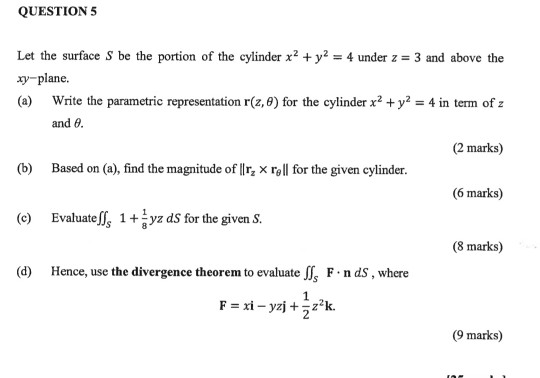QUESTION 5 Let the surface S be the portion of the cylinder x2 + y2 4 under z 3 and above the xy-plane Write the parametric representation r(z,0) for the cylinder x2 +y2 4 in term of z (a) and 0 (2 marks) Based on (a), find the magnitude of llr, x rell for the given cylinder (b) (6 marks) 1 1+ (e) Evaluate z dS for the given S (8 marks) Hence, use the divergence theorem to evaluate f, F.n ds, where (d) 1 Fxi-yzjz2k. 2 (9 marks)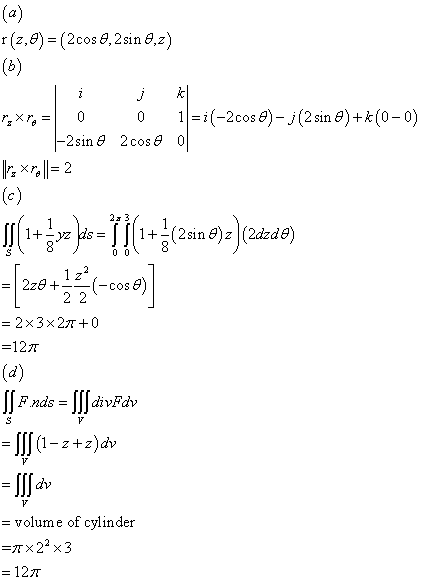##### Add Answer of: QUESTION 5 Let the surface S be the portion of the cylinder x2 + y2 4 under z 3 and above the xy-plane Write the par...
Similar Homework Help Questions
• ### 5. Calculate the surface area of the portion of the sphere x2+y2+12-4 between the planes z-1 and z ะไ 6. Evaluate (xyz) dS, where S is the portion of the plane 2x+2y+z-2 that lies in the first oc...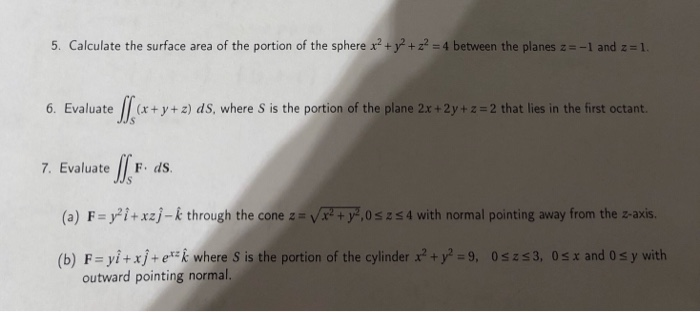5. Calculate the surface area of the portion of the sphere x2+y2+12-4 between the planes z-1 and z ะไ 6. Evaluate (xyz) dS, where S is the portion of the plane 2x+2y+z-2 that lies in the first octant. 7. Evaluate F. ds. a) F = yli + xzj-k through the cone z = VF+ア0s z 4 with normal pointing away from the z-axis. b) F-yi+xj+ek where S is the portion of the cylinder+y9, 0szs3, 0s r and O s y...

• ### Could you do number 4 please. Thanks 1-8 Evaluate the surface integral s. f(x, y, z) ds Vx2ty2 -vr+) 1. f(x, y, z) Z2; ơ is the portion of the cone z between the planes z 1 and z 2 1 2. f(x, y, z)...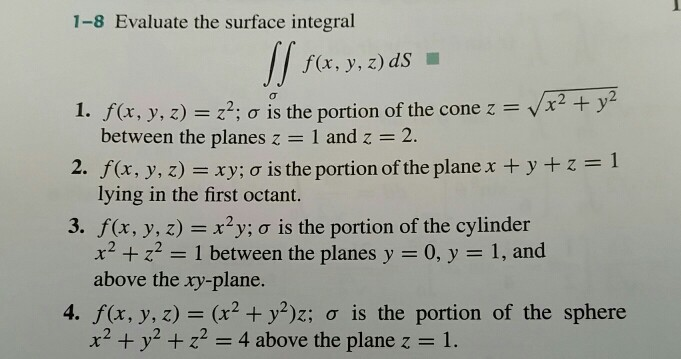Could you do number 4 please. Thanks 1-8 Evaluate the surface integral s. f(x, y, z) ds Vx2ty2 -vr+) 1. f(x, y, z) Z2; ơ is the portion of the cone z between the planes z 1 and z 2 1 2. f(x, y, z) xy; ơ is the portion of the plane x + y + z lying in the first octant. 3. f(x, y, z) x2y; a is the portion of the cylinder x2z2 1 between the planes...

• ### 7. Evaluate z ds. where s is the surface z-= x2 + y2, x2 + y2-1.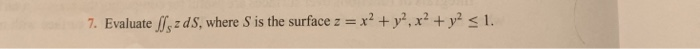7. Evaluate z ds. where s is the surface z-= x2 + y2, x2 + y2-1. 7. Evaluate z ds. where s is the surface z-= x2 + y2, x2 + y2-1.

• ### Let S be part of the paraboloid ~ = x2 + y2 that lies under the plane ž Evaluate the surface inte...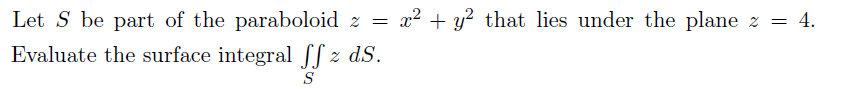Subject: Calculus 3 Let S be part of the paraboloid ~ = x2 + y2 that lies under the plane ž Evaluate the surface integral [z d.S 4. Let S be part of the paraboloid ~ = x2 + y2 that lies under the plane ž Evaluate the surface integral [z d.S 4.

• ### Let E-xi vi + 2zk be an electrostatic field. Use Gauss's Law to find the total charge enclosed by the closed surface consisting of the hemisphere- V1-x2 - y2 and its circular base in the xy-plane...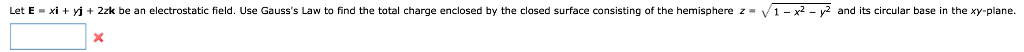Let E-xi vi + 2zk be an electrostatic field. Use Gauss's Law to find the total charge enclosed by the closed surface consisting of the hemisphere- V1-x2 - y2 and its circular base in the xy-plane. Use the Divergence Theorem to evaluate F.N dS and find the outward flux of F through the surface of the solid bounded by the graphs of the equations. Use a computer algebra system to verify your results F(x, y, z) =xyì + 7yj +xzk...

• ### 1, Evaluate Jsx2ds where s is the portion of the cylinder 22ヤU-9 lying between z-1 and 22. 2. Let F(, y, z)i+ j + zk....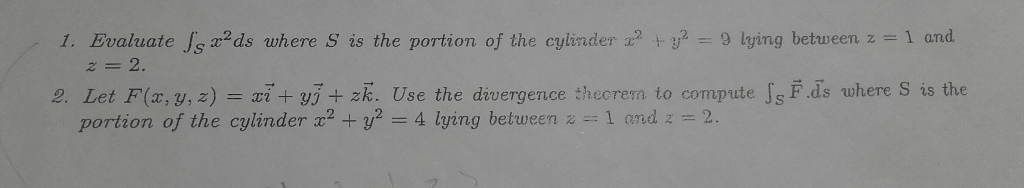1, Evaluate Jsx2ds where s is the portion of the cylinder 22ヤU-9 lying between z-1 and 22. 2. Let F(, y, z)i+ j + zk. Use the divergence thecrem to compute s F.ds where S is the portion of the cylinder x2 +y2-4 lying between z 1 and-2. 1, Evaluate Jsx2ds where s is the portion of the cylinder 22ヤU-9 lying between z-1 and 22. 2. Let F(, y, z)i+ j + zk. Use the divergence thecrem to compute s...

• ### Evaluate 1 dS where s is the surface z = 3 inside the cylinder x2 + y2-1. B.π C. 3/2 D. 2T E. 3π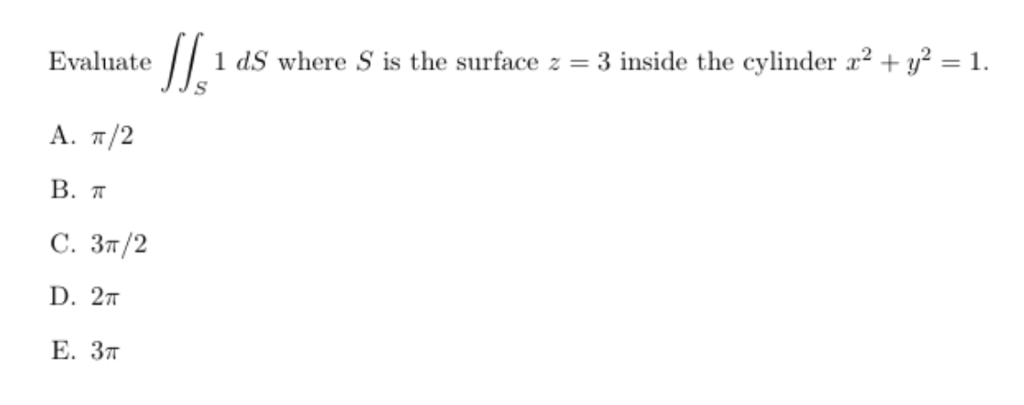Evaluate 1 dS where s is the surface z = 3 inside the cylinder x2 + y2-1. B.π C. 3/2 D. 2T E. 3π Evaluate 1 dS where s is the surface z = 3 inside the cylinder x2 + y2-1. B.π C. 3/2 D. 2T E. 3π

• ### Let E be the solid that lies inside the cylinder x^2 + y^2 = 1, above the xy-plane, and below the...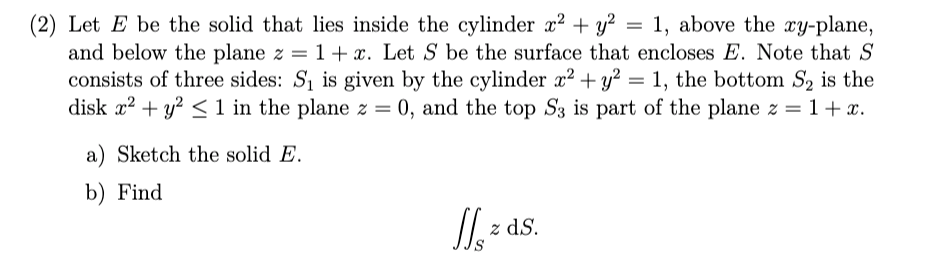Let E be the solid that lies inside the cylinder x^2 + y^2 = 1, above the xy-plane, and below the plane z = 1 + x. Let S be the surface that encloses E. Note that S consists of three sides: S1 is given by the cylinder x^2 + y^2 = 1, the bottom S2 is the disk x^2 + y^2 ≤ 1 in the plane z = 0, and the top S3 is part of the plane z...

• ### 1 point) Find the volume of the wedge-shaped region (Figure 1) contained in the cylinder x2 + y2-4 and bounded above by the plane z = x and below by the xy-plane. z=x FIGURE 1 1 point) Find the...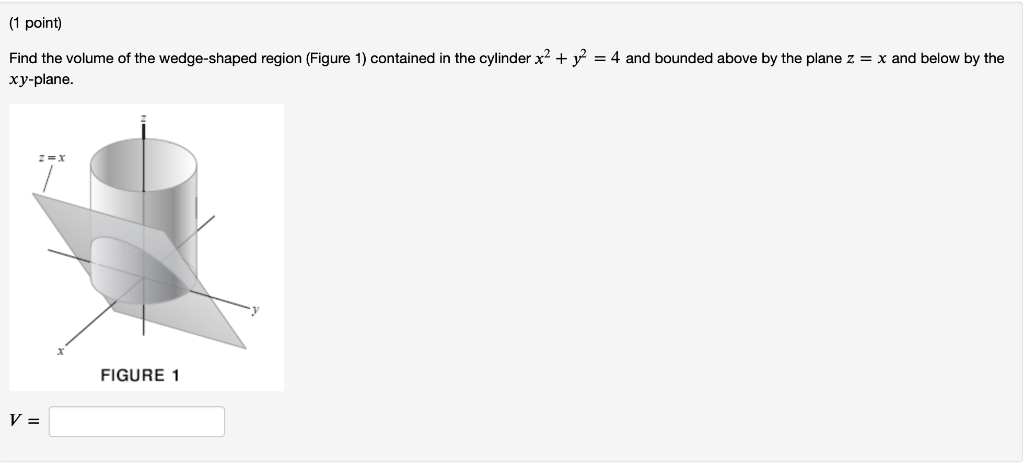1 point) Find the volume of the wedge-shaped region (Figure 1) contained in the cylinder x2 + y2-4 and bounded above by the plane z = x and below by the xy-plane. z=x FIGURE 1 1 point) Find the volume of the wedge-shaped region (Figure 1) contained in the cylinder x2 + y2-4 and bounded above by the plane z = x and below by the xy-plane. z=x FIGURE 1

• ### 9. Let Q be the solid bounded by the cylinder x2 + y2 = 1 and the planes z = 0 and z = 1 . Use the Divergence Theorem to calculate | | F . N dS where s is the surface of Q and F(x, y, z) = xi + yj +...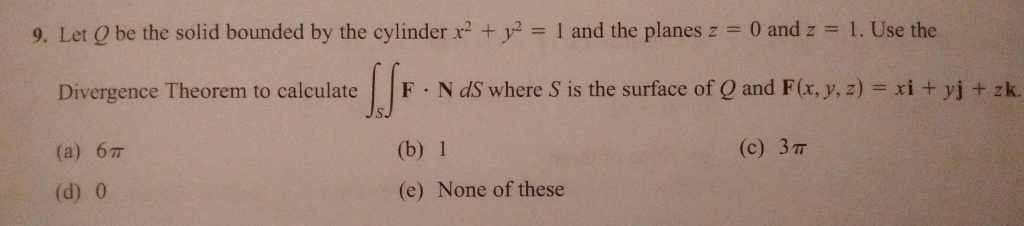9. Let Q be the solid bounded by the cylinder x2 + y2 = 1 and the planes z = 0 and z = 1 . Use the Divergence Theorem to calculate | | F . N dS where s is the surface of Q and F(x, y, z) = xi + yj + zk. (a) 67T (d) 0 (b) 1 (e) None of these (c) 3π 9. Let Q be the solid bounded by the cylinder x2 + y2...

Need Online Homework Help?